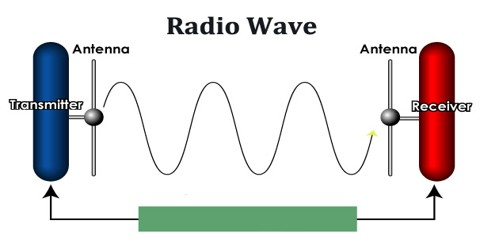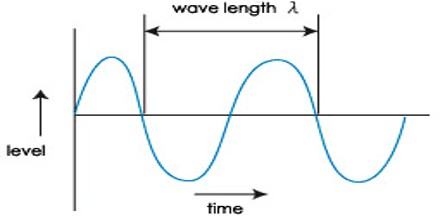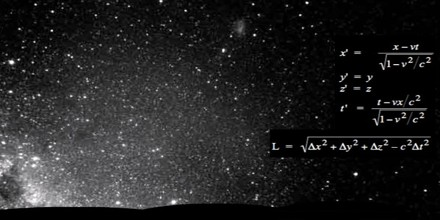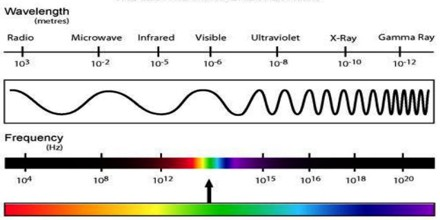PhysicsDefinition

Radio Wave is a very low frequency electromagnetic wave (from roughly 30 kilohertz to 100 gigahertz). Radio-wave communications signals travel through the air in a straight line, reflect off of clouds or layers of the ionosphere, or are relayed by satellites in space. They are used in standard broadcast radio and television, short-wave radio, navigation and air-traffic control, cellular telephony, and even remote-controlled toys.Electromagnetic radiation is transmitted in waves or particles at different wavelengths and frequencies. This broad range of wavelengths is known as the electromagnetic (EM) spectrum. The spectrum is generally divided into seven regions in order of decreasing wavelength and increasing energy and frequency. The common designations are radio waves, microwaves, infrared (IR), visible light, ultraviolet (UV), X-rays and gamma-rays.

Radio waves have the longest wavelengths in the EM spectrum, according to NASA, ranging from about 1 millimeter (0.04 inches) to more than 100 kilometers (62 miles). They also have the lowest frequencies, from about 3,000 cycles per second or 3 kilohertz (kHz) up to about 300 billion hertz, or 300 gigahertz (GHz).Radio waves travel at the speed of light. When passing through an object, they are slowed according to that object’s permeability and permittivity.

Standing Waves – The amplitude of the wave decreases as 1/r, where r is the distance from the transmitter. At the point of reflection, the incident and reflected waves have the same amplitude, but elsewhere the incident wave has larger amplitude than the reflected one. Thus the nodes in the combined wave do not have amplitude of zero (except at the point of reflection); rather, they are local minima in the interference pattern.

As we saw in interference, the nodes are separated by half a wavelength, which is here L = λ/2 = 0.50 m. The oscilloscope setting had 2 ns per division, giving 20 ns across the screen. The period of the wave is measured at T = 3.3 ns (the oscillator was set at 300 MHz), so the speed is λ/T = 3.0 X 108 m.s−1.

It’s interesting to compare this measured speed of radio waves with the measured speed of light. Maxwell noted that the speed of the wave solution to the equations of electromagnetism was similar to that measured for light. This was persuasive evidence that light was an electromagnetic wave.Light, electromagnetism, time and space – As mentioned above, the speed of electromagnetic radiation, c = √ (1/ε0μ0), appears as the characteristic speed in Maxwell’s equations of electromagnetism. It also appears in the theory of relativity, where it is the natural conversion between time and space. In space-time, the separation betwen two events (with separations of space Δx, Δy, Δz and in time Δt) is given by

L = √ (Δx2 + Δy2 + Δz2 − c2Δt2).

Electromagnetic radiation travels through space without a medium. When Lamour, Lorentz, Fitzgerald and Einstein proposed this, however, this relation was much less obvious.Wavelength and Frequency of Radio Waves

The wavelength is the distance from one peak of the wave’s electric field (wave’s peak/crest) to the next, and is inversely proportional to the frequency of the wave. The distance a radio wave travels in one second, in a vacuum, is 299,792,458 meters (983,571,056 ft) which is the wavelength of a 1 hertz radio signal. A 1 megahertz radio signal has a wavelength of 299.8 meters (984 ft).